The eqns. (2.53) and (2.54) contain several gradients of scalar and vectorial functions which will be evaluated in this section. The following two identities which represent the gradients of a scalar- and a vector-field are helpful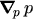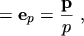(2.55)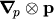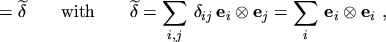(2.56)

where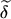is the unity tensor and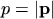.

The calculation of the gradients of the weight functions of even order is straightforward: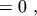(2.57)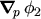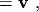(2.58)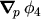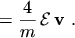(2.59)

The calculation of the gradients of the weight functions of odd order takes into account that eqns. (2.49) to (2.51) all have the same functional form,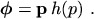(2.60)

Applying the product rule and using eqns. (2.55) and (2.56) yields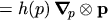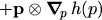(2.61)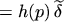(2.62)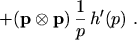(2.63)

The derivatives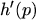are readily obtained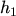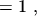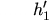(2.64)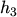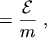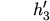(2.65)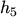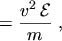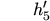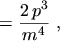(2.66)

which finally allows the gradients of the odd weight functions to be written as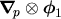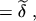(2.67)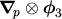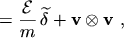(2.68)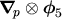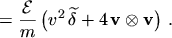(2.69)

M. Gritsch: Numerical Modeling of Silicon-on-Insulator MOSFETs PDF